# 自定义函数场景案例 Demo ​

## 开通创建自定义函数 ​

JavaScript
``````/**
* 方法名必须为 codec
* 出参：任意类型的数据，不能为空
*/

if( t >= -50 && t <= 50){
json["temperature"] = t.toFixed(2) + '°C';
}else{
json["temperature"] = "error";
}

if( h >= 0 && h <= 100){
json["humidity"] = parseInt(h) + '%';
}else{
json["temperature"] = "error";
}

return json;
}``````
``````/**
* 方法名必须为 codec
* 出参：任意类型的数据，不能为空
*/

if( t >= -50 && t <= 50){
json["temperature"] = t.toFixed(2) + '°C';
}else{
json["temperature"] = "error";
}

if( h >= 0 && h <= 100){
json["humidity"] = parseInt(h) + '%';
}else{
json["temperature"] = "error";
}

return json;
}``````

## 调用自定义函数 ​

sql
`````` SELECT
FROM
"temp_hum/pub/test"``````
`````` SELECT
FROM
"temp_hum/pub/test"``````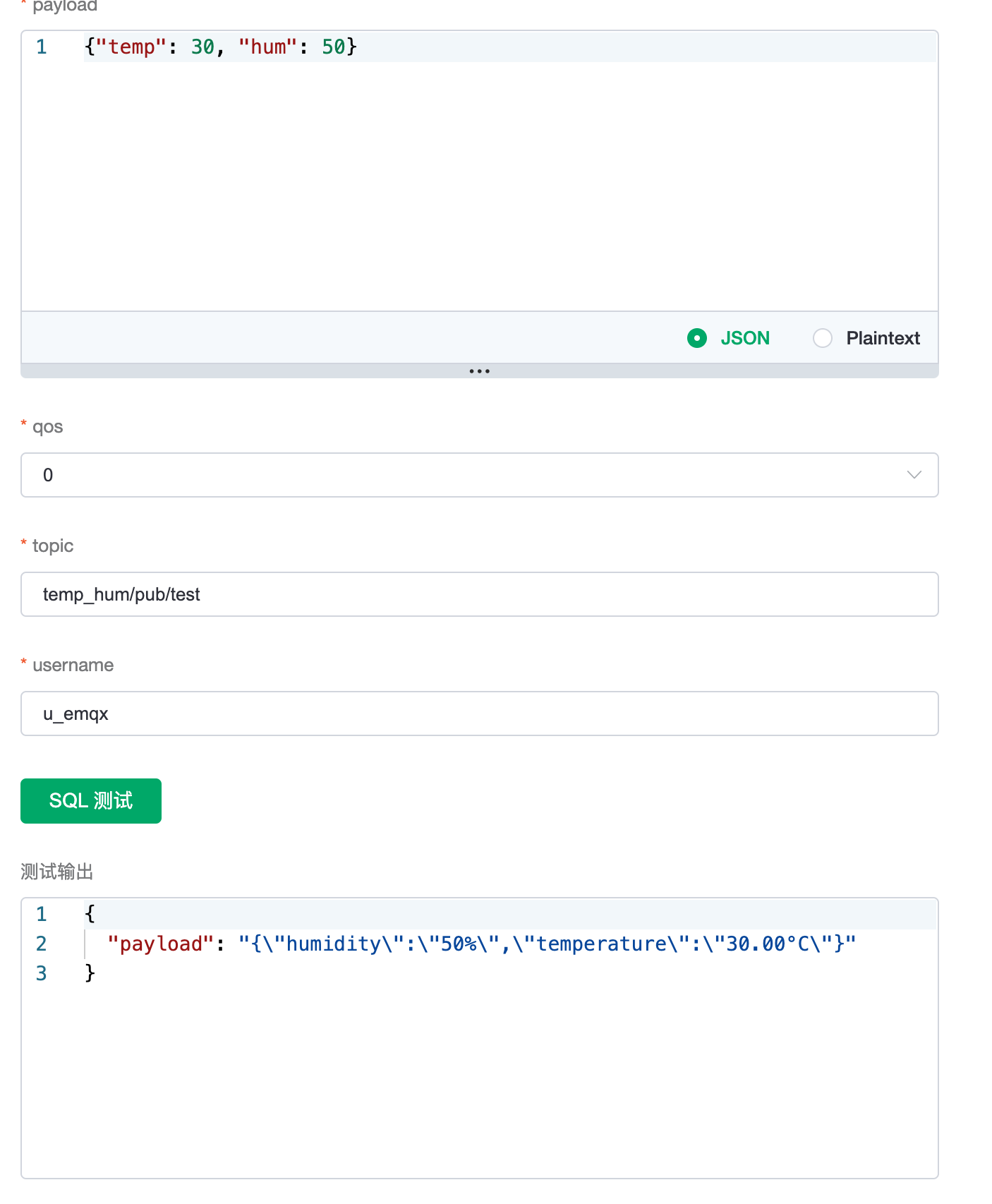## 连接到客户端发送消息 ​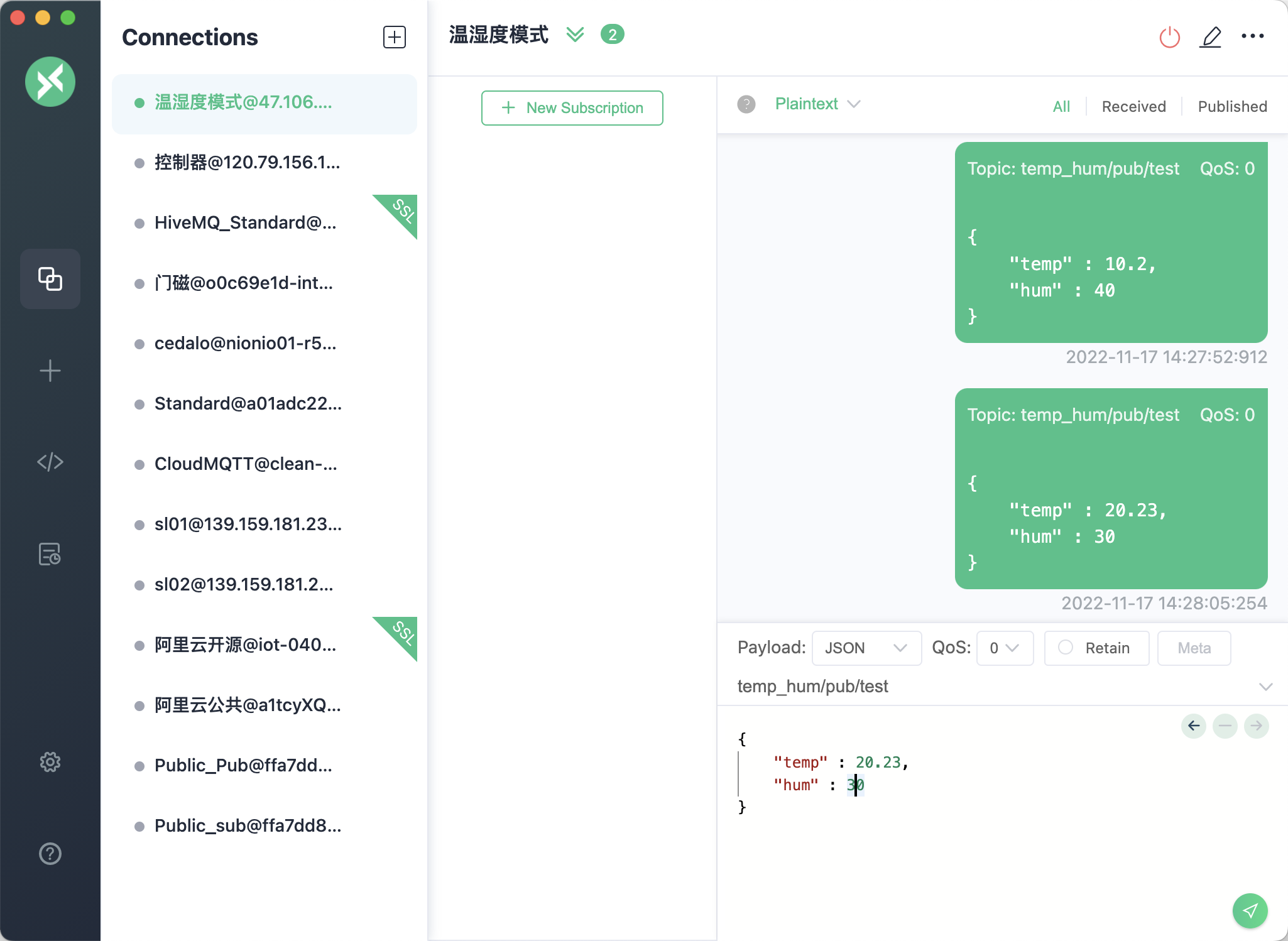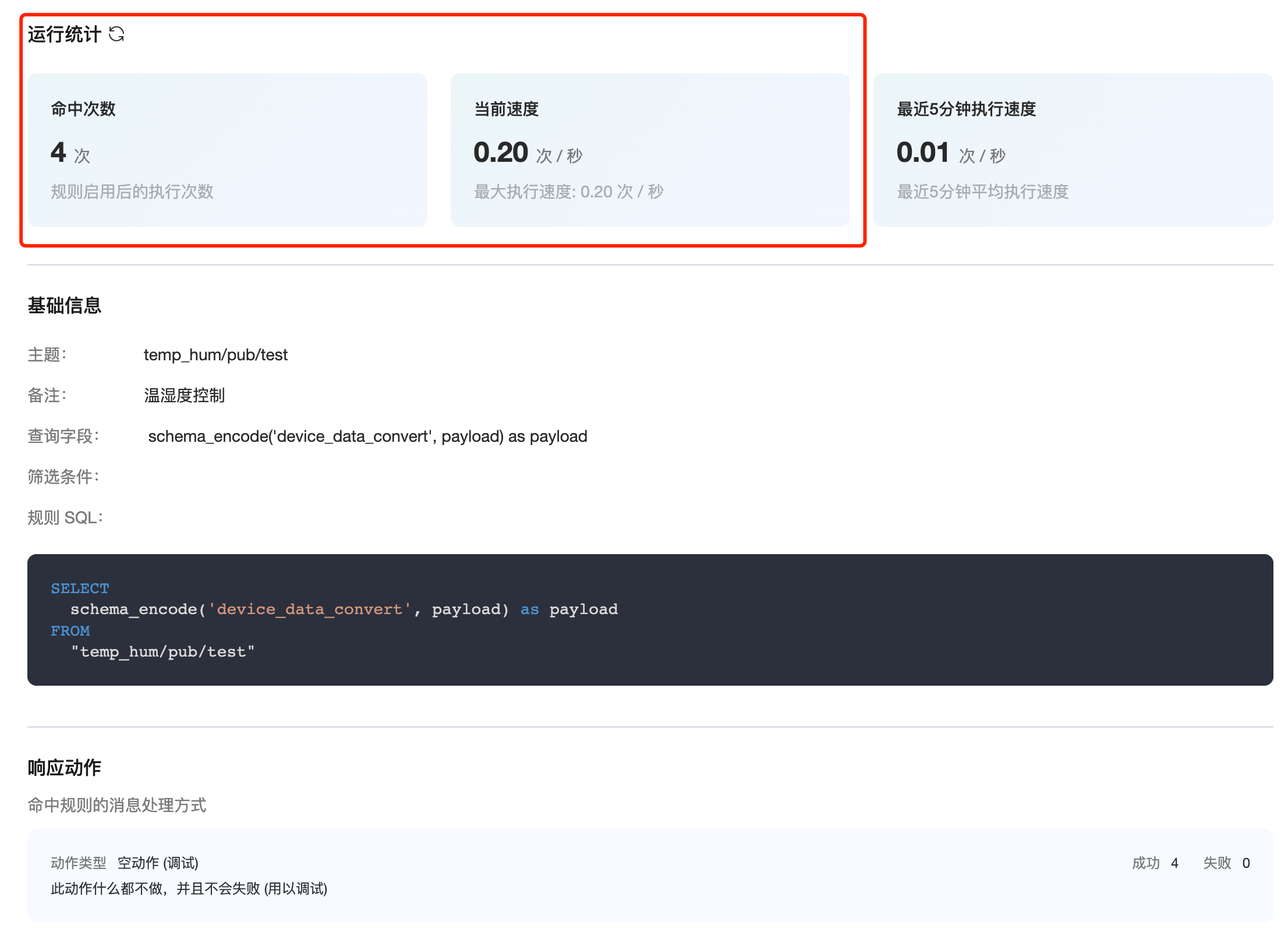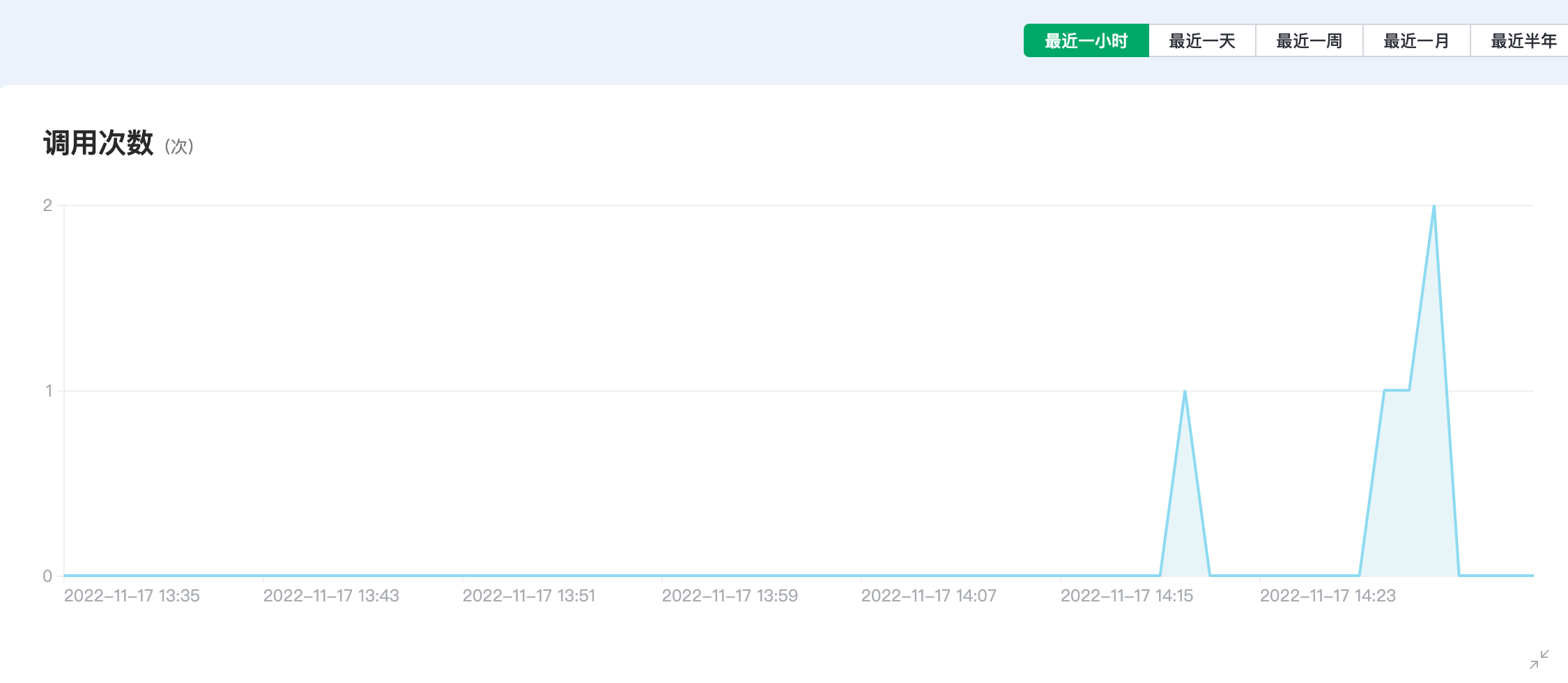JavaScript
``````/**
* 方法名必须为 codec
* 出参：任意类型的数据，不能为空
*/

if( t >= -50 && t <= 50){
json["temperature"] = t.toFixed(2) + '°C';
if(t >= 40) {
}
}else{
json["temperature"] = "error";
}

if( h >= 0 && h <= 100){
json["humidity"] = parseInt(h) + '%';
}else{
json["temperature"] = "error";
}

return json;
}``````
``````/**
* 方法名必须为 codec
* 出参：任意类型的数据，不能为空
*/

if( t >= -50 && t <= 50){
json["temperature"] = t.toFixed(2) + '°C';
if(t >= 40) {
}
}else{
json["temperature"] = "error";
}

if( h >= 0 && h <= 100){
json["humidity"] = parseInt(h) + '%';
}else{
json["temperature"] = "error";
}

return json;
}``````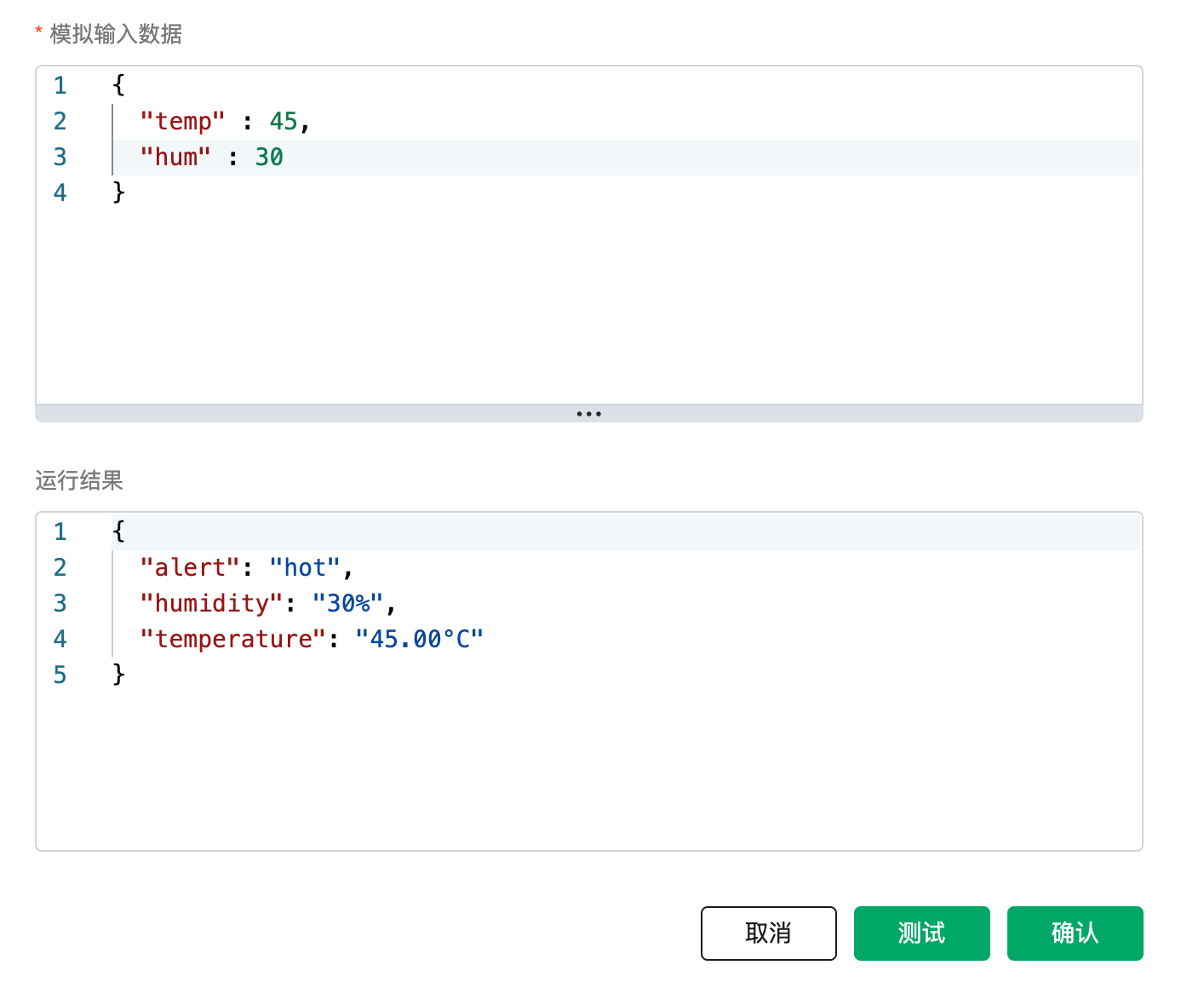sql
`````` SELECT
`````` SELECT